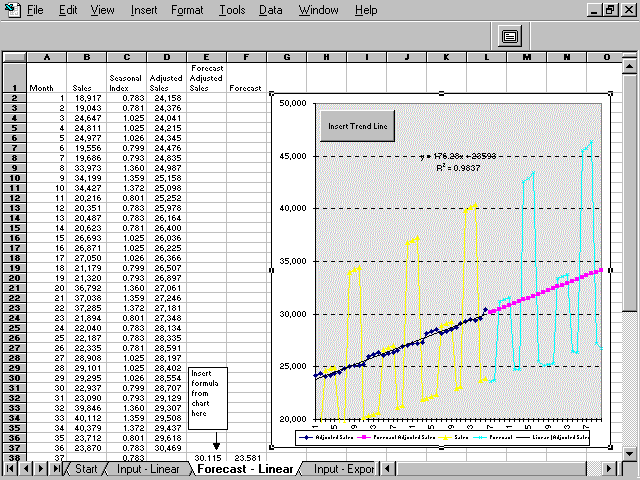## Forecasting Techniques

Keywords: Forecasting, Trend line, Linear Regression, Statistics, Sales, Time Series

Description: Example of applying Excel's forecasting features to the process of forecasting sales. This method provides the user with a method of eliminating seasonal trend and the R^2 statistic to compare the fit of various shape curves to the adjusted data.

Tip: You can use Excel's built-in forecasting features to create a sales forecast. Use the Average formula and use moving averages to estimate seasonal indices.

Adjust the actual data with the seasonal indices and plot the resulting data. Use Excel's trendline feature to add a best fit line to the chart. This workbook contains an example of a linear and polynomial sales trend. You can compare trendline R^2 values to find the best fit. R^2 is percent of variation explained by trendline -- Higher R^2 values are best -- 1=perfect fit.

After finding the best fit, use the trendline formula and seasonal indices to forecast future periods.

Guide to the Example:
1) Enter Sales in column B of Input Data Sheet
2) Recreate trendline on chart in Forecast sheet - Activate chart and click on Insert Trendline button. Choose best fitting trendline (linear, ploynomial, etc.) by comparing R^2 value.
3) Replace formula in cell E38 with formula from trendline and copy down column E -- see examples.